# Learning Electronics

Learn to build electronic circuits

# Phasor mathematics

### Question 1:

Provide a definition for phasor, as the term applies to electrical calculations.
A "phasor" is a complex-number representation of an electrical quantity, such as voltage, current, or impedance.

Notes:
The ingredient of complex must be present in any definition of a phasor. A phasor, while it may be classified as a type of vector (possessing both magnitude and direction), is not the same as the vectors commonly used in other areas of physics (e.g. force vectors, electric/magnetic field vectors, etc.).

### Question 2:

A series is a very important concept in mathematics. The common notation for a ßeries" looks something like this (a type of "geometric" series is shown here as an example):

 �� n=0 xn

Explain what this notation means, and how we may approximate the value of a series for a finite value of n.
A ßeries" is comprised of a set of terms added together, each term related to the others by a common form, distinguished only by the incrementing or decrementing of a common variable. To illustrate by example, here is an expansion of the geometric series shown in the question:

 �� n=0 xn = x0 + x1 + x2 + x3 + x4 + �+ x�

Follow-up question: what do you think the value of this series would be if the value of x was greater than (positive) 1? What if x = 0? What if 0 < x < 1?

Notes:
The purpose of this question is to familiarize students with the concept of a mathematical series, and the notation used to describe series.

### Question 3:

A common mathematical function known as factorial is represented by an exclamation point following a positive integer number. The following are all examples of factorials:

 1! = 1          2! = 2          3! = 6          4! = 24          5! = 120          6! = 720

Explain in your own words what this "factorial" function represents. What procedure (algorithm) would we use to arithmetically calculate the value of any factorial given to us?
I'll let you research this on your own! The algorithm isn't that difficult to figure out just by examining the sequence of factorials given in the question.
Follow-up question: calculate 0!

Notes:
In addition to being very useful in probability calculations, factorials are frequently seen in important mathematical series, including those used to calculate e, sin, and cos.

### Question 4:

Euler's constant, that ubiquitous constant in mathematics symbolized by the letter e, may be found as the result of the following mathematical series:

 e = �� n=0 1 n!

Approximate the value of e in steps, using the following table:

 n n! 1 / n! � e 0 1 2 3 4 5 6 7

Also, express this series as a partial sum up to n = 7.

 n n! 1 / n! � e 0 1 1 1 1 1 1 2 2 2 0.5 2.5 3 6 0.16667 2.66667 4 24 0.04167 2.70833 5 120 0.00833 2.71667 6 720 0.00139 2.71806 7 5040 0.00020 2.71825

Shown here is the partial sum up to n=7:

 1 0! + 1 1! + 1 2! + 1 3! + 1 4! + 1 5! + 1 6! + 1 7!

. . . and again, in slightly different form . . .

 1 + 1 + 1 2 + 1 6 + 1 24 + 1 120 + 1 720 + 1 5040

Notes:
It should go without saying that students should consult their electronic calculators (or a textbook) to see what the actual value of e is, and compare that to their partial sum approximation.
This questions provides students with the opportunity to see where e comes from, and also to explore the behavior of a series by calculating partial sums. Many students find it fascinating to see the sequence of partial sums converge on the true value of e, seeing how this formerly mysterious constant can actually be calculated using nothing more than repeated arithmetic.

### Question 5:

The trigonometric function of cosine may be found as the result of an infinite series. Note that this series assumes the angle x to be expressed in units of radians, not degrees:

 cosx = �� n=0 (-1)n x2n (2n)!

Approximate the cosine of 1 radian (cos1) in steps, using the following table, then write the partial sum expansion up to n = 5:

 n (-1)n x2n (2n)! � cosx 0 1 2 3 4 5

 n (-1)n x2n (2n)! � cosx 0 1 1 1 1 1 -1 1 2 0.5 2 1 1 24 0.5416667 3 -1 1 720 0.5402778 4 1 1 40320 0.5403026 5 -1 1 3628800 0.5403023

Shown here is the partial sum expansion up to n = 5:

 cosx � 1 - x2 2! + x4 4! - x6 6! + x8 8! - x10 10!

Notes:
It should go without saying that students should consult their electronic calculators to see what the actual value of cos1 is, and compare that to their partial sum approximation. Students should also feel free to explore the validity of this series by approximating the cosine of angles other than 1 radian.
This questions provides students with the opportunity to see how cosx may be arithmetically calculated. This, in fact, is how many digital electronic computers determine trigonometric functions: from partial sum approximations.

### Question 6:

The trigonometric function of sine may be found as the result of an infinite series. Note that this series assumes the angle x to be expressed in units of radians, not degrees:

 sinx = �� n=0 (-1)n x2n+1 (2n+1)!

Approximate the sine of 1 radian (sin1) in steps, using the following table, then write the partial sum expansion up to n = 5:

 n (-1)n x2n+1 (2n+1)! � sinx 0 1 2 3 4 5

 n (-1)n x2n+1 (2n+1)! � sinx 0 1 1 1 1 1 -1 1 6 0.8333333 2 1 1 120 0.8416667 3 -1 1 5040 0.8414683 4 1 1 362880 0.8414710 5 -1 1 39916800 0.8414710

Shown here is the partial sum expansion up to n = 5:

 sinx � x - x3 3! + x5 5! - x7 7! + x9 9! - x11 11!

Challenge question: which series (sine or cosine) would a computer be able to calculate quickest, given a certain number of digits (precision) past the decimal point? In other words, which of these two infinite series converges fastest?
Shown here is a partial sum expansion of the cosine function, for comparison:

 cosx � 1 - x2 2! + x4 4! - x6 6! + x8 8! - x10 10!

Notes:
It should go without saying that students should consult their electronic calculators to see what the actual value of sin1 is, and compare that to their partial sum approximation. Students should also feel free to explore the validity of this series by approximating the sine of angles other than 1 radian.
This questions provides students with the opportunity to see how sinx may be arithmetically calculated. This, in fact, is how many digital electronic computers determine trigonometric functions: from partial sum approximations.

### Question 7:

Compare these three mathematical series, one for ex, one for cosx, and one for sinx:

 ex = 1 + x + x2 2! + x3 3! + x4 4! + x5 5! + x6 6! + x7 7! + �

 cosx = 1 - x2 2! + x4 4! - x6 6! + �

 sinx = x - x3 3! + x5 5! - x7 7! + �

Note similarities in the terms of these three series. Aside from signs, it would appear the cosine series contains all the even-powered terms of ex and the sine series contains all the odd-powered terms of ex. Leonhard Euler, the Swiss mathematician who lived from 1707 to 1783, found a connection between these three series which became known as Euler's relation.
You too can find this same connection if you substitute jx for x in the first (ex) series, and then multiply all terms in the sine series by j. Leave the cosine series unaltered. Remember that j = {-1} and that j2 = -1, j3 = -j, j4 = 1, j5 = j, etc.
First, I'll perform the substitutions and multiplications for you:

 ejx = 1 + jx + (jx)2 2! + (jx)3 3! + (jx)4 4! + (jx)5 5! + (jx)6 6! + (jx)7 7! + �

 cosx = 1 - x2 2! + x4 4! - x6 6! + �

 j sinx = jx - j x3 3! + j x5 5! - j x7 7! + �

If you properly calculate all the powers of j, you will find this relationship between these three series:

 ejx = cosx + j sinx

Notes:
For those students who need a little more help, here is the ejx series before and after simplification:

 ejx = 1 + jx + (jx)2 2! + (jx)3 3! + (jx)4 4! + (jx)5 5! + (jx)6 6! + (jx)7 7! + �

 ejx = 1 + jx - x2 2! - j x3 3! + x4 4! + j x5 5! - x6 6! - j x7 7! + �

Now, the composition of the ejx series as being the sum of the cosx series and the j sinx series should be more evident.

### Question 8:

A fascinating mathematical identity discovered by Leonhard Euler (1707-1783), regarded by some as the most beautiful equation in all of mathematics, relates five of mathematics' fundamental constants together:

 ei p + 1 = 0

Use Euler's relation to translate this identity into trigonometric terms, where the truth of this identity will become more evident.

 cosp+ j sinp+ 1 = 0

Notes:
The beauty of this identity cannot be denied, relating five fundamental constants of mathematics (e, i, p, 1, and 0) together in one simple equation.
Note: here I break stylistic convention by using the more traditionally mathematical i instead of the traditionally electrical j to represent {-1}. For those who just can't stand to see i represent anything other than instantaneous current, here you go:

 ej p + 1 = 0

Are you happy now?

### Question 9:

If you have studied complex numbers, you know that the same complex quantity may be written in two different forms: rectangular and polar. Take for example the complex quantity [(3)/2] + j1/2. The following illustration shows this point located on the complex plane, along with its rectangular dimensions: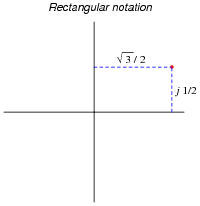Next, we see the same point, on the same complex plane, along with its polar coordinates: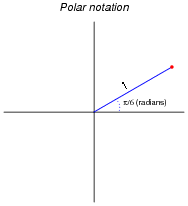Written out, we might express the equivalence of these two notations as such:

 �3 2 + j 1 2 = 1 � p 6

Expressed in a more general form, the equivalence between rectangular and polar notations would look like this:

 a + jb = c �Q

However, a problem with the ängle" symbol () is that we have no standardized way to deal with it mathematically. We would have to invent special rules to describe how to add, subtract, multiply, divide, differentiate, integrate, or otherwise manipulate complex quantities expressed using this symbol. A more profitable alternative to using the ängle" symbol is shown here:

 a + jb = c ejQ

Explain why this equivalence is mathematically sound.
The equivalence shown is based on Euler's relation, which is left to you as an exercise to prove.

Notes:
This question should probably be preceded by #04058, which asks students to explore the relationship between the infinite series for ex, cosx, and sinx. In any case, your students will need to know Euler's relation:

 ejx = cosx + j sinx

### Question 10:

Electrical engineers usually express the frequency of an AC circuit in terms of angular velocity, measured in units of radians per second rather than cycles per second (Hertz, or Hz).
First, explain what a radian is. Next, write an equation relating frequency (f) in Hertz to angular velocity (w) in radians per second. Hint: the relationship between the two is perhaps most easily understood in terms of a two-pole AC generator, or alternator, where each revolution of the rotor generates one full cycle of AC.
A radian is that angle describing a sector of a circle, whose arc length is equal to the radius of the circle: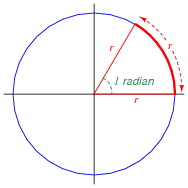Next, the equivalence between angular velocity (w) and frequency (f):

 w = 2 pf

Notes:
Personally, I find the rotating alternator model the best way to comprehend the relationship between angular velocity and frequency. If each turn of the rotor is one cycle (2 p radians), and frequency is cycles per second, then one revolution per second will be 1 Hertz, which will be 2 p radians per second.

### Question 11:

Suppose we have a simple two-pole AC generator, or alternator, the two stator windings on either side of the rotor connected together so as to function as a single winding: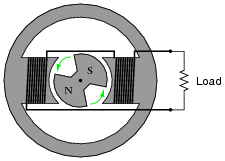Ideally, this machine will generate a sinusoidal output voltage as the rotor turns. Suppose now that we write index marks on the rotor shaft to measure its position as it turns, and we represent that position with the Greek letter "Theta" (Q). It is purely arbitrary where we label the "zero" position on the shaft, so we choose to mark that point at some easily-identified point on the output waveform: the place where the coil output voltage peaks positive while rotating counter-clockwise. When spun, the instantaneous output voltage will then match the cosine function. In other words, the instantaneous output voltage will be proportional to the cosine of the shaft angle: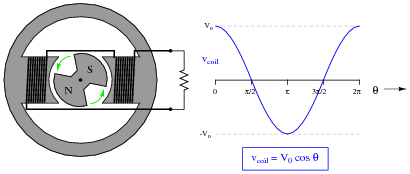We may represent the coil's voltage with the equation vcoil = V0 cosQ. If we precisely know the peak voltage value (V0) and the shaft position (Q), we may precisely predict the coil voltage at any instant in time (vcoil).
However, there is a more complete way of describing what is happening in this alternator. The equation vcoil = V0 cosQ is adequate for predicting coil voltage from shaft position, but it is not adequate for doing the reverse: predicting shaft position from coil voltage. Note that there are only two points on the voltage waveform where a voltage value corresponds to a unique shaft position, and those points are the positive and negative peaks. At any other value of instantaneous voltage (-V0 < vcoil < V0), there are multiple possible shaft positions. The most obvious example of this is where vcoil = 0. Here, the shaft position could be [(p)/2] radians, or it could be [(3 p)/2] radians. Note that we consider the positive peak to occur at only one position (0 radians) and not two positions (0 and 2 p radians) in one revolution because 2 p radians is identical to 0 radians just as 360 degrees is equivalent to 0 degrees.
In order to uniquely describe the alternator's shaft position in terms of output voltage, we need more information than just the instantaneous voltage at one coil. What we need is another coil, an imaginary coil, shifted in angular position from the first coil: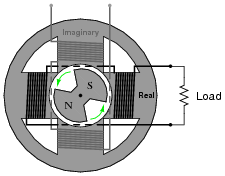Like the first coil (the real coil), the imaginary coil's output voltage will also be sinusoidal. However, it will generate an output voltage at a different phase than the real coil's output voltage.
Plot the waveform of the imaginary coil's voltage, superimposed on the waveform of the real coil's output voltage, and then write an equation expressing both instantaneous voltages as a complex sum: the real coil's voltage as a real number and the imaginary coil's voltage as an imaginary number (complete with the j prefix). Then, use Euler's relation to re-write this complex sum as a complex exponential expression.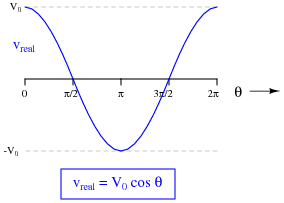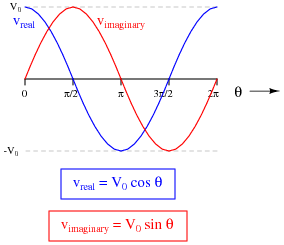Alternator output as a complex sum:

 Vout = V0 cosQ+ j V0 sinQ

Alternator output as a complex exponential:

 Vout = V0 ej Q

Notes:
Here, I tried my best to give simple, real-world meaning to phasor notation. Interestingly, the oft-lamented label of ïmaginary" actually works to my advantage, describing the output of a coil that has no useful purpose but to define the alternator's shaft position in terms of a quadrature voltage.

### Question 12:

 �f(x)  dx   Calculus alert!

Impedance is defined as the complex ratio of voltage and current:

 Z = V I

We may determine the complex definition of impedance provided by an inductor if we consider the equation relating inductor voltage and inductor current:

 v = L d dt [ i(t) ]

First, substitute a phasor expression of current into the above equation, then differentiate with respect to time to obtain an expression for voltage:

 i(t) = ej wt

 v = L d dt [ ej wt ]

Then, divide the two phasor expressions to obtain an expression for inductive impedance.

 ZL = j wL

Follow-up question: explain why the following expression for inductive impedance is equivalent to the one shown above:

 ZL = wL  ej p/ 2

Notes:
In order to solve this problem, your students must remember the basic rule for differentiating exponential functions:

 d dx [ eax ] = a eax

This is one of the beauties of representing sinusoidal voltages and currents in complex exponential (phasor) form: it makes differentiation and integration relatively easy! In this sense, the Euler relation of ejx = cosx + j sinx is a transform function, transforming one type of mathematical problem into a different (easier) type.

### Question 13:

 �f(x)  dx   Calculus alert!

Impedance is defined as the complex ratio of voltage and current:

 Z = V I

We may determine the complex definition of impedance provided by an capacitor if we consider the equation relating capacitor voltage and capacitor current:

 i = C d dt [ v(t) ]

First, substitute a phasor expression of voltage into the above equation, then differentiate with respect to time to obtain an expression for current:

 v(t) = ej wt

 i = C d dt [ ej wt ]

Then, divide the two phasor expressions to obtain an expression for capacitive impedance.

 ZC = 1 j wC or          ZC = -j 1 wC

Follow-up question: explain why the following expression for capacitive impedance is equivalent to the one shown above:

 ZC = 1 wC ej (-p/ 2)

Notes:
In order to solve this problem, your students must remember the basic rule for differentiating exponential functions:

 d dx [ eax ] = a eax

This is one of the beauties of representing sinusoidal voltages and currents in complex exponential (phasor) form: it makes differentiation and integration relatively easy! In this sense, the Euler relation of ejx = cosx + j sinx is a transform function, transforming one type of mathematical problem into a different (easier) type.